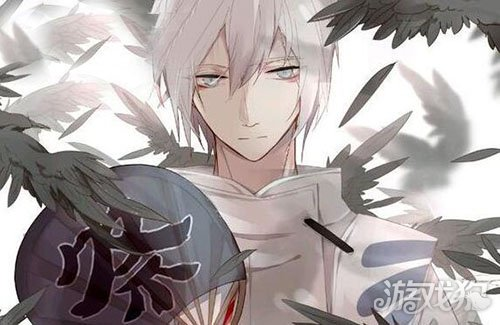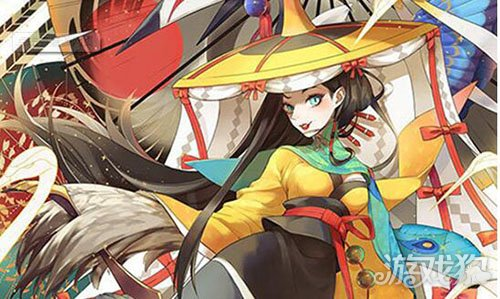# 阴阳师大天狗和姑获鸟哪个好对比说明

2017-01-11 16:42:10来源：游戏狗整理编辑：阿熳1W血的小怪

2W的小怪 (包括大蛇身边17000血的大天狗)

3.5W的中间怪

10W的大蛇

6804*1.2= 8164 5600 =13764

8316*1.1=9147*1.2=10976 5600 =16576

4428*1.1=4870*1.2=5844 4200 =10044

6804*1.2= 8164 6300*2*0.8*1.2*1.2=14515 =22679

8316*1.1=9147*1.2=10976 6000*2*0.8*1.2*1.2=13824 =24800

4428*1.1=4870*1.2=5844 6000*2*0.8*1.2*1.2=10368 =16212

7500 攻击 100%暴击 160爆伤

-----------------------------------

20000

7800*0.6*2.31*1.6=13478 针女4000 = 17478

7500*0.6*2.31*1.6*1.1=18295 针女4000 = 22295

7500*0.6*1.23*1.6*1.1=9742 针女3000 = 12742

---------------------------------------

35000

7800*0.6*2.31*1.6=13478 针女7000 = 20478

7500*0.6*2.31*1.6*1.1=18295 针女7000 = 25295

7500*0.6*1.23*1.6*1.1=9742 针女5250 = 14992

---------------------------------------

100000

7800*0.6*2.31*1.6=13478 针女20000 = 31348

7500*0.6*2.31*1.6*1.1=18295 针女20000 = 38295

7500*0.6*1.23*1.6*1.1=9742 针女15000 = 24742

(当怪物血量分布是10W 1.7W 1.7W的时候，谁都知道打完10W血boss的效率就是你的总效率)